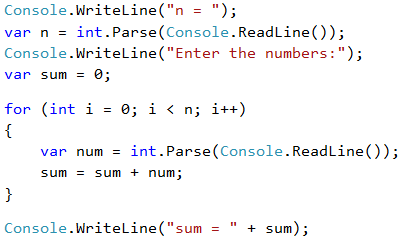Problem: Summing up Numbers

Write a program that inputs n integers and sums them up.

• The first line of the input holds the number of integers n.
• Each of the following n lines holds an integer for summing.
• Sum up the numbers and finally print the result.

Input Output
2
10
20
30
3
-10
-20
-30
-60
4
45
-20
7
11
43
1
999
999
0 0

Video: Summing Numbers

Watch this video to learn how to sum numbers in for-loop: https://youtu.be/t7PAichwl7k.

Hints and Guidelines

We can solve the problem with summing up numbers in the following way:

• We read the input number n.
• We run a loop from 1 to n. On each step of the loop, we read the number num and add it to the sum sum.
• Finally, we print the calculated amount sum.

Below is the source code for the solution: